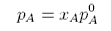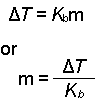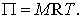##### ch. 13 physical properties of solutions

0
Set Details Share
created 11 years ago by tlk2074
127 views
concentration units
updated 11 years ago by tlk2074
College: First year, College: Second year, College: Third year, College: Fourth year
Subjects:
chemistry
Page to share:
Embed this setcancel
COPY
code changes based on your size selection
Size:
X
1

Solution

homogeneous mixture of 2 or more substances
solid, liquid, or gas
uniform composition
"like dissolves like"

2

Solvent is ________ amount and solute is a __________ amount.

solvent is larger
solute is smaller

3

Polar dissolves ________ or _______. But not ___________

polar or ionic
not non-polar

4

Sugar and water dissolve well because they form ________ bonds with one another

hydrogen

5

Types of concentration units

percent by mass
molarity
molality
mole fraction

6

Crystallization

process in which dissolved solute comes out of solution and forms crystals

7

Percent by mass

% by weight

mass of solute
------------- * 100
total mass of solution

8

Molarity

(M) number of moles of solute in 1L of solution
- can be changed by temperature

Moles of solute
---------------
liters of solution

9

Molality

(m) number of mold of solute dissolved in 1 kg (1000g) of solvent

moles of solute
-------------
mass of solvent (kg)

10

Mole Fraction

(x)

moles of solute
-------------
total moles of solution

11

3 types of saturations

Saturated solutions
supersaturated
unsaturated

12

Supersaturated

contains more solute than is preset in a saturated solution

13

Saturated

maximum amount of a solute in a given solvent

14

Unsaturated

less solute than it has capacity to dissolve

15

Concentration

amount of solute present in given solution

16

Amount to use for conversions using Mass Percent

100.0 g of solution

17

Amount to use for conversions using Molality

1 kg of solvent

18

Amount to use for conversions using Molarity

1L of solution

19

Amount to use for conversions using Mole Fraction

1 total mole

20

Henry's Law

the solubility of a gas in a liquid is proportional to the the pressure of the gas

C=kP

K depends on particular gas (mol/L*atm)
P- in atm
C- molar concentration (moles/L) of dissolved gas

21

Solubility

the maximum amount of a solute that will dissolve in a given quantity of solvent at a specific temperature

22

For solids and liquids, in most situations, solubility _____ as temperature ________

increases
increases

23

In gases, solubility ________ when temperature __________

decreases
increases

24

Colligative Properties

several important properties of solutions depend on the number of solute particles in the solution and not on the nature of the solute particles.

bound together by a common origin

they depend on the number of solute particles present whether these partoc;es are atoms, ions, or molecules

25

Types of colligative properties

-vapor pressure lowering
-boiling point elevation
-freezing point depression
-osmotic pressure

26

nonvolitle solute

doesn not have a measurable vapor pressure

27

Vapor pressure loweringnonvolitle with solvent
sugar molecules inhibits water from evaporating
decrease in vapor pressure= increase in concentration

Pa= vapor pressure
Xa=Mole fraction
P*a= pure solvent

28

Boiling Point Elevationnonvolitle solute lowers vapor pressure - affect boiling point

at any temperature the vapor pressure of the solution is lower than that of the pure solvent

29

Freezing Point depression

Make sure to subtract from normal freezing point
method of thawing succeeds because it depresses the freezing point of water

deltaTf = Kfm

deltaTf- freezing point of depression
Kf- molal freezing point depression constant
m- molality

30

Osmotic Pressurethe pressure required to stop osmosis. Represented by Pi
M=Molarity (mol/L)
T= Absolute Temp (K)
Pi= Osmotic Pressure (atm)

31

meaning of i

for electrolytes, "i" should be added to an equation

Actual number of particles in a solution after dissociation
i= --------------------------------------------------
number of formula units initially dissolved in solution

32

Formula to find mole fraction of water (x1)

N1
X1= -------
N1 + N2